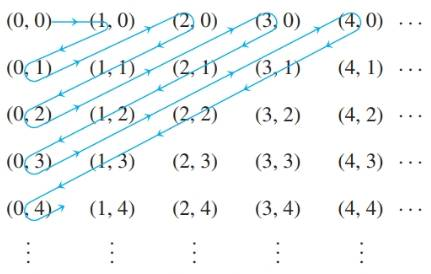Chapter 7.4, Problem 23ES### Discrete Mathematics With Applicat...

5th Edition
EPP + 1 other
ISBN: 9781337694193

#### Solutions

Chapter
Section### Discrete Mathematics With Applicat...

5th Edition
EPP + 1 other
ISBN: 9781337694193
Textbook Problem
1 views

# âa. Explain how to use the following diagram to show that Z n o n n e g × Z n o n n e g and Z n o n n e g have the same cardinality.b. Define a function H :   Z n o n n e g × Z n o n n e g → Z n o n n e g by the formula H ( m ,   n ) = n + ( m + 1 ) ( m + n + 1 ) 2 for all nonnegative integers m and n. Intercept the action of H geomatrically using the diagram of part (a).

To determine

(a)

To explain the following diagram to show that Znonveg×Znonveg and Znonveg cardinality-based problem.

Explanation

To proof Znonveg×Znonveg has the same cordinality as Znonveg

Let f: Znonveg ( Znonveg×Znonveg, f(x)=xth value in the path created in diagram .Thus more precisely:

f(0)=( 0,0)f(1)=( 1,0)f(2)=( 2,0)f(3)=( 1,1)f(4)=( 1,1)f(5)=( 0,2)f(6)=

To determine

(b)

To explain the following diagram to show that and cardinality based problem

### Still sussing out bartleby?

Check out a sample textbook solution.

See a sample solution

#### The Solution to Your Study Problems

Bartleby provides explanations to thousands of textbook problems written by our experts, many with advanced degrees!

Get Started